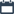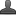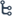## Thursday, October 15, 2020

•October 15, 2020
••, ,
•No comments

## Introduction to Co-Ordinate Geometry (10th class )Chapter-7,Definition,Concept,Important points,Choose the correct answer Bits

Co-Ordinate Geometry

Hi friends and my dear students! In this post, I have covered Andhra Pradesh class 10Introduction to Co-Ordinate Geometry (10th class ) chapter-7 important points After Reading Mathematics Co-Ordinate Geometry Notes With important points, please do share it with your friends. You can Learn Maths for All Classes here.

IMPORTANT POINTS

* To locate the exact position of a point on a number line we need only a single reference.

* To describe the exact position of a point on a Cartesian plane we need two references.

* Rene Descartes a French mathematician developed the new branch of mathematics called Co-ordinate Geometry.

* The two perpendicular lines taken in any direction are referred to as co-ordinate axes.

* The horizontal line is called X- axis.

*The vertical line is called Y- axis.

* The meeting point of the axes is called the origin.

* The distance of a point from Y-axis is called the x co-ordinate or abscissa.

* The distance of a point from X-axis is called the  y  co-ordinate or ordinate.

* The co-ordinates of origin are (0, 0).

*The co-ordinate plane is divided into four quadrants namely Q1, Q2, Q3, Q4, i.e., first, second, third, and fourth quadrants respectively.

* The signs of co-ordinates of a point are as follows

. Q;1: (+, +)    Q2: (- ,+)    Q3: (- , -)      Q4: (+, -)

* The x co-ordinate of a point on Y- axis is zero

* The   y co-ordinate of a point on X - axis is zero.

* Equation of X- axis is y = 0

* Equation of Y - axis is x = 0

* In a co-ordinate plane (x1, y1) #(x2, y2) unless   x1 = x2 and   y1 = y2Also Check

Introduction to Knowing Our Numbers Key Points

## Trigonometry Do This & Try this solution

SSC(10th class) Trigonometry Exercise - 11.1 Solutions

1.To locate the exact position of a point, the number of references we need is      (  D )

A) 0            B) 1          C) 4                 D) 2

2. The quadrant in which the point (-1,9) lies is           (   C   )

A) Q4         B) Q3        C) Q2        D) Q1

3. The point (0, - 9) lies                                        (   B   )

A)  On x-axis B) on Y-axis   c) in Q3    D) in Q4

4. The quadrant in which the point (-3, -9) lies is          (   B   )

A) Q4         B) Q3        C) Q2        D) Q1

5. The quadrant in which the point (4, -7) lies is          (   B   )

A) Q4         B) Q3        C) Q2        D) Q1

6. What is the general form of the points which lie on x-axis? (   A   )

A)(x, o)       B) (o, x)              C) (x, y)       D) (y, o)

7. In a co-ordinate system the horizontal   line is called    (  C   )

A) Y-axis       B) Origin       C) X-axis        D) Reference

8. The meeting point of the two axes in the co-ordinate plane is called   (  D    )

A) Y-axis    B) X-axis      c) (2, 3)   D) Origin

9. A point on the X-axis is              ( D  )

A) (2, 5)       B) (0, 5)      C) (3, 3)       D) (9, 0)

10. A point on the Y-axis is            (   D   )

A) (4,-2) C) (4, 4) B) (-8, 3) D) (0, 1)

11. In the C0-ordinate plane the axes are       (  B    )

... to each other.

A) parallel     B) perpendicular   C) neither parallel no perpendicular  D) none

12. The co-ordinates of origin are                 (   C   )

A) (1, 1)      B) (- 1, 1)      C) (0, 0)      D) (1, 0)

13. The point (- 2, - 7) lies in           (   D   )

A) Q2         B) Q4        C) Q 1      D) Q3

14. The point which is not on the X-axis          (   D   )

A)  (- 1, 0)              B) (7, 0)      C) (2, 0)          D) (0, 8)

15. The points (0, 0), (0, 3), (4, 3) and (4, 0) are plotted in graph sheet. The figured formed by joining the points with    straight lines is            (  D   )

A) Triangle     B) square        C) circle      D) rectangle

16. The point which lies on both the axes is     (   D    )

A) (1, 1)       B) (2, 2)      C) (-1, - 1)       D) (0, 0)

17. X-axis is denoted by                   (   A    )

A) y = 0         B) x = 0       C) x = y     D) none

18. Y-axis is denoted by                                  (   B    )

A) y = 0        B)  x = 0           C)x = y        D)all the above

19. If the distance of a point from X-axis is 3 units and its distance from Y-axis 9units   then the point is denoted by    (   D    )

A) (8, 3)       B) (-8, 3)          C) (-3, 9)       D) (3, 9)

20. A (2, 5) and   B (3, 7) are points in a graph sheet. The ordinate of B is more than     that of A by         (   B   )

A) 1                  B) 2           C) 3                      D) 4

21. The mathematician who found an association between algebraic equations and geometric curves and figures is          (   A   )

A) Rene Descartes     B) Euclid     C) John Venn      D) Archimedes

22. The y- co-ordinate of the point A (-4, -3) exceeds its x- co-ordinate by    (   c   )

A) - 1            B) - 7         C) 1    D) 7

23. The number of quadrants that the co- ordinate axes divide the Cartesian plane     into is            (   D   )

A) 1            B)   2          C)   3   D) 4

24. Which of the following points is at a distance of 6 units from Y - axis measured along positive X-axis?         (   B   )

A) (8, 6)     B)  (6, 8)  C) (8, 2)     D) (8, 6)

25. The x - co-ordinate of (4, 5) is called               (   A   )

A) Abscissa      B) ordinate     C) direction       D) 4

26. The y - coordinate of (6, - 8) is called               (   C   )

A) Abscissa       B) - 8             C) ordinate          D) locations

27. Which of the following is on OX in Graph?      (   A   )

A) (1, 0) B) (0, 1) C) (0, 0) D) (0, - 1)

28. If x > 0, y > 0, then (x, y) lies in             (   D    )

A) Q3     B) Q1    C) Q4    D) Q,

29. If x < 0, y < 0, then (x, y) line is              (   B   )

A) Q4     B) Q3    C) Q2   D) Q1

30. If x > 0, y < 0, than     (x, - y) lies in       (   C    )

A) Q3       B) Q2     C) Q1        D) Q4

31. If x >0, y < 0, then (-x, y) lies in             (   C   )

A) Q1     B) Q2    C) Q3     D) Q

32. The point with abscissa 3 and whose ordinate is less than its abscissa by 4  (  D  )

A) (3, 4)           B) (- 1, - 2)             C) (-1, 2)          D) (-1, 3)

33. The point which lies in Q4   is              (   B   )

A) (1, 2)          B) (1,-2)        C) (-3, 4)       D) (3, - 1)

34. Which of the following is on OY graph?         (   B   )

A) (4, 0)       B) (0, 0)             C) (-3, 0)         D) (-3, - 2)

35. Which of the following point’s abscissa is more than its ordinate by 2?   (   D   )

A) (- 4, 2)          B ) (- 1, 1)     C) (2, 4)             D) (1, - 1)

36. The figure formed with vertices (0, 0), (1, 0), (1, 1) and (0, 1) is called a    (   B   )

A) rectangle       B) square      C) circle      D) none

37. The point (-3, 0) lies on              (   B   )

A) OX             B) OX'       C) OY       D) OY1

38. The quadrant in which the point (1, 2) lies is      (   D   )

A) Q4         B) Q3        C) Q2        D) Q1

39. The abscissa of the point (-7, 9) is                  (   A    )

A)-7             B) 9             C) 7                     D) -5

40. The ordinate of the point (4, - 9) is                 (   C   )

A) 4     B) – 2     C)-9         D) 2

41. The abscissa and ordinate of a point- 3 and- 4 respectively. Hence the co-ordinates of the point are   (   B   )

A) (- 2, 4)     B) (-3, - 4)     C) (3, - 4)     D) (3, - 4)

42. Which of the following points lie on the axis?             (   A    )

A) (8, 0)    B) (- 2, – 3) C) (4, – 2) D) (-1, 4)

43. The quadrant in which the point.  (5, – 6) lies is       (   D    )

A) Q1    B) Q2       C) Q3    D) Q4

44. The quadrant in which the point (- 8, 9) lies is          (   C   )

A) Q4         B) Q3        C) Q2     D) Q1

45. The point which lies in Q3 is                                      (   D   )

A) (- 7, 4)    B) (0, 4)   C) (2, 0)    D) (-2, – 6)

46. The number of references needed to locate the exact position a point in a plane is             (   C   )

A) 1              B) 4               C) 2.           C) 3

47. The quadrant in which the point (- x, - y) lies where x < 0, y < 0 is       (   C    )

A) Q4            B) Q,                 C) Q,             D) Q3

48. The point which lies on both the axes is                    (   D    )

A) (-5, -8)       B) (-1, 4)     C) (2, - 3)           D) (0, 0)

49. The point which is not on the Y-axis is                       (   A   )

A) (- 3, 0)      B) (0, 3)      C) (0,- 3)       D) (0, 4)

50. The   X -CO-ordinate of a point is also called           (   C   )

A) Ordinate     B) CO-ordinate   C) abscissa   D) none

51. The point (0, - 3) lies on                                            (   D    )

A) OX        B) OX'     C) OY         D) OY1

52. The point (- 3, 2) lies in - - - - - - quadrant.      (   C   )

A) IV           B) III          C) II         D) I

53. The distance of a point from Y-axis is called            (  C   )

A) y co-ordinate   B) 2nd co-ordinate   C) abscissa   D) origin

54.The mathematician who developed   the study of co-ordinate geometry is (  D    )

A) Euler       B) John Venn       C) Euclid        D) Rene Descartes

55. The distance of a point from X-axis is called         (   D   )

A) x co-ordinate C) first co-ordinate D) ordinate B) abscissa

56. In a co-ordinate system the vertical line is called    ( A  )

A) Y-axis       B) Origin       C) X-axis        D) Reference